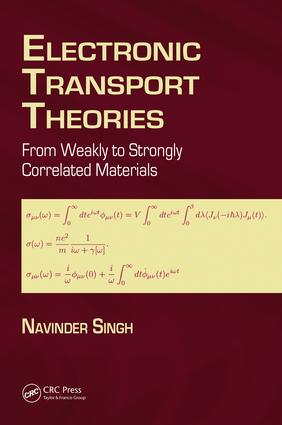# Electronic Transport Theories

## From Weakly to Strongly Correlated Materials, 1st Edition

CRC Press

213 pages | 38 B/W Illus.

##### Purchasing Options:\$ = USD
Hardback: 9781498743594
pub: 2016-10-26
SAVE ~\$37.00
\$185.00
\$148.00
x
eBook (VitalSource) : 9781315368962
pub: 2016-11-17
from \$28.98

FREE Standard Shipping!

### Description

Maintaining a practical perspective, Electronic Transport Theories: From Weakly to Strongly Correlated Materials provides an integrative overview and comprehensive coverage of electronic transport with pedagogy in view. It covers traditional theories, such as the Boltzmann transport equation and the Kubo formula, along with recent theories of transport in strongly correlated materials. The understood case of electronic transport in metals is treated first, and then transport issues in strange metals are reviewed. Topics discussed are: the Drude-Lorentz theory; the traditional Bloch-Boltzmann theory and the Grüneisen formula; the Nyquist theorem and its formulation by Callen and Welton; the Kubo formalism; the Langevin equation approach; the Wölfle-Götze memory function formalism; the Kohn-Luttinger theory of transport; and some recent theories dealing with strange metals. This book is an invaluable resource for undergraduate students, post-graduate students, and researchers with a background in quantum mechanics, statistical mechanics, and mathematical methods.

### Table of Contents

Introduction and objective of the study. From Drude-Lorentz to Sommerfeld-Bloch and from Bloch-Peierls to Kubo-Holstein: A historical introduction. Optical absorptivity and reflectivity: brief experimental background. The Drude model at finite frequencies for simple metals. The Lorentz model for simple insulators. Brief discussion of the optical properties of real metals and real insulators.Summary points. Bibliography. The traditional Boltzmann equation based approaches. Semiclassical model of electron dynamics. Chambers’ method for Boltzmann kinetic equation (relaxation time approximation). Beyond the relaxation time approximation (full Boltzmann equation). The Bloch-Boltzmann transport theory. Summary points. Bibliography. Some techniques from nonequilibrium statistical mechanics. Nyquist’s thermodynamical arguments and Callen-Welton’s fluctuation-dissipation theorem. Kubo’s formalism. The Drude formula from the Einstein relation. The Drude formula from the Langevin equation. Problem of the Langevin equation. The generalized Langevin equation and the memory function (time dependent friction coefficient). When can one use the exponential decay of the velocity-velocity correlation function?. Summary points. Bibliography. The Zwanzig-Mori-Gotze-Wolfle memory function formalism. The Zwanzig-Mori memory function (MF) formalism. The Gotze-Wolfle (GW) formalism. Summary points. Bibliography. The Kohn-Luttinger theory: Quantum mechanical basis of the Bloch-Boltzmann equation. The assumptions in the traditional kinetic equations. The problems with the assumptions. The model and assumptions. The formalism. Why and how are the traditional kinetic theories justified?. Summary points. Bibliography. Strange metals: A survey. General introduction to the problem. A survey of the experimental situation. A survey of the theoretical situation. Bibliography. Electronic transport theories from simple to strange metals: A summary. Bibliography. Supporting material and practice exercises. Appendix A: Proving f∗μν (ω) = fνμ(ω). Appendix B: Computing h(|H′kk′ |2)2iimp. Appendix C: The concept of quasiparticles. Practice exercises.

### About the Author

Navinder Singh is a faculty member of the Physical Research Laboratory, Ahmedabad. He received a B.E. in electronics, and henceforth shifted to the field of theoretical physics. He obtained his Ph.D. in theoretical condensed matter physics from the Raman Research Institute, Bangalore, from 2000-2006. He then obtained his post doctoral training from IOP Bhubaneswar; Holon Institute of Technology, Holon, Israel; and the University of Toronto, Canada. His research interests include electronic transport in strongly correlated systems like strange metals, and the theory of (un)conventional superconductivity.

### Subject Categories

##### BISAC Subject Codes/Headings:
SCI055000
SCIENCE / Physics
SCI077000
SCIENCE / Solid State Physics
TEC021000
TECHNOLOGY & ENGINEERING / Material Science